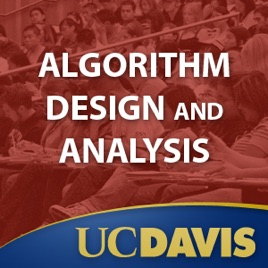30 episodes

The purpose of this undergraduate course is to introduce fundamental techniques and viewpoints for the design and the analysis of efficient computer algorithms, and to study important specific algorithms. The course relies heavily on mathematics and mathematical thinking in two ways: first as a way of proving properties about particular algorithms such as termination, and correctness; and second, as a way of establishing bounds on the worst case (or average case) use of some resource, usually time, by a specific algorithm. The course covers some randomized algorithms as well as deterministic algorithms.# Algorithm Design and Analysis UC Davis

• Technology

The purpose of this undergraduate course is to introduce fundamental techniques and viewpoints for the design and the analysis of efficient computer algorithms, and to study important specific algorithms. The course relies heavily on mathematics and mathematical thinking in two ways: first as a way of proving properties about particular algorithms such as termination, and correctness; and second, as a way of establishing bounds on the worst case (or average case) use of some resource, usually time, by a specific algorithm. The course covers some randomized algorithms as well as deterministic algorithms.

4.1 out of 5
10 Ratings

10 Ratings